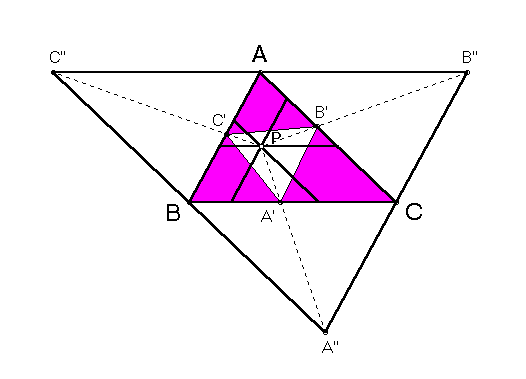# EQUAL PARALLELIANS POINT

 There is a point P in the plane of an arbitrary triangle ABC such that the three segments through P parallel to the sidelines of ABC and having endpoints on these sidelines have equal lengths. Perhaps the earliest mention of this point occurs in one of Peter Yff's notebooks, written in 1961. Yff determined that the ratio of lengths of the segments into which P separates the BC-parallelian is (bc + ca - ab) : (ab + bc - ca), so that the product of the three ratios along the three parallelians is 1. (More generally, the point common to any three concurrent parallelians separates them in ratios with product 1.) Trilinear coordinates for the equal parallelians point are bc(ca + ab - bc) : ca(ab + bc - ca) : ab(bc + ca - ab), where a, b, c are the sidelengths of triangle ABC.(For a quick lesson on trilinears, click on TRILINEAR COORDINATES.)

Triangle Centers